output.to from Sideway
Draft for Information Only

# Mechanics: Statics

Statics is the study of forces which act on a rigid body with no change in momentum occur and is concerned with the static equilibrium state of the rigid body under the action of forces as stated in Newton's First and Third Laws. But static mechanics is mainly deal with problems of statics of which rigid bodys are always at rest.

Force

By Newton's Second Law, the accelarion of an object is directly proportional to the applied force and inversely proportional to the object mass. Applied force is measured as 1 Newton when the applied force cause an object of mass 1 kg to accelerae at 1m/s and can be expressed F=ma. As the object is also accelerated according to the direction of the applied force, force is a vector quantity. But, in fact, the nature of a force is derived from the resultant of interaction between two objects.

Idealizations

Idealizations are techniques used to simplify the application of theory in problem modeling. They are

• Particle is a point mass and its size can be neglected

• Rigid Body is one single object formed by a combination of particles in which all particles remain at a fixed distance from one another and are assumed to be rigid under applied forces.

• Concentrated Force is a mathematical representation of the effect of forces acting on a rigid body by assuming a single force acts at a point on the object along a single line of force in space.

Types of Force

The most common types of forces are

• Gravity force: Gravity force is the volume force dealing with the Newton's law of gravitational attraction. The earth exerts an gravitational force of weight downward on every objects on its surface. Gravity force is also called body force or weight of an object.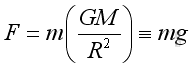and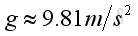• Surface Force: Surface force is force applied to the surface of an object.

• Applied Force: Applied force is a surface force acting upon an object. The total force applied by a surface force can be deposited to normal force and pallarel force with respect to the surface of the object.

• Normal Force: Normal Force is force applied to the suface of an object perpendicularly. It can be a compression force to squash an object or an extension force to strench an object.

• Friction: Friction is the force applied to the surface of an object parallelly by a surface due to the interaction between two surfaces. There are two types of friction.

• Force deals with two at rest object surfaces relative to each other is static friction. Static friction force is the force resisting object motion. Before motion begins, the static friction force is of equal magnitude and opposite direction of the net applied force. The maximum possible static friction between two surfaces is the product of the normal force between two surfaces and the coefficient of static friction of the two surfaces involved.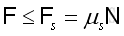• Force deals with two moving object surfaces relative to each other is kinetic friction. The direction of friction force is opposite to the motion. When motion begins, the kinetic friction force can be approximated by the product of the normal force between two surfaces and the coefficient of kinetic friction of the two surfaces involved. The kinetic friction force acts as the opposite force to retard the motion.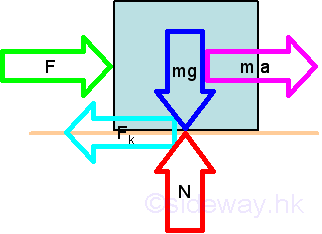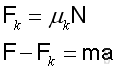• Tension: Tension is force exerted on a rope or a cable when pulling on. The applied force is transmitted from one end to the other along the rope.  It is similar to the extension force of stretching an object.

ID: 110100001 Last Updated: 27/1/2014 Revision: 3 Ref:References

1. F.P. Beer; E.R. Johnston,Jr.; E.R. Eisenberg, 2004, Vector Mechanics for Engineers: Statics
2. I.C. Jong; B.G. rogers, 1991, Engineering Mechanics: Statics and Dynamics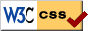Nu Html Checker53na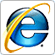naHome 5

Management

HBR 3

Information

Recreation

Culture

Chinese 1097

English 337

Computer

Hardware 149

Software

Application 198

Digitization 118

Numeric 19

Programming

Web 283

Unicode 494

HTML 65

CSS 58

ASP.NET 96

OS 389

Python 19

Knowledge

Mathematics

Algebra 25

Geometry 18

Calculus 67

Engineering

Mechanical

Rigid Bodies

Statics 92

Dynamics 37

Control

Physics

Electric 27